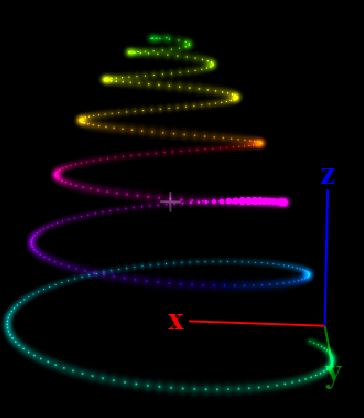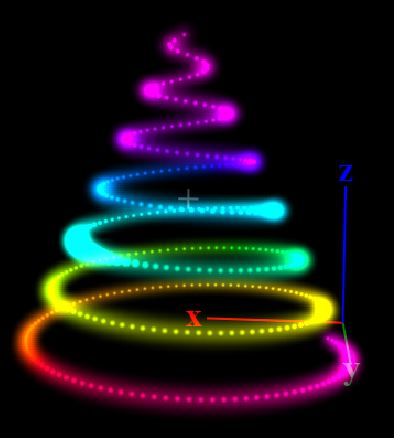# Spiral Christmas Tree

Hi guys,

I have PixelBlaze in my christmas tree. Have some LEDs in a sprial way up the tree. Wanted to map. 1st manuall, but that seems overkill. Tried to do a simple sprail, but that would render to much of the pixels close to the top.

Did some googling and found a good tipp on stackoverflow. Put everything into one piecce of code for your convenience.

Key advantage is that the LEDs are äquidistant over the whole string.

``````function (pixelCount) {

var n = 3;
var R = 1.0;
var H = 1.0

var s = Math.PI * 2 * n
var map = [];

for (i = pixelCount; i > 0; i--) {
b = i + 1
t = 2 * Math.PI * Math.sqrt(s / b / pixelCount )
r = R - b * t
map.push([Math.cos(i * t) * r , Math.sin(i * t) * r, H - H/pixelCount * i])
}
return map
}
``````

You might find this usefull.

5 Likes

Thank you for posting this mapping. I’m going to help a friend with her tree tomorrow, and it was very useful for me. I changed one thing about the pattern because the Z scaling as presented makes the vertical distance between spiral rings closer at the top of the tree. I scaled the height of each LED with the distance to the tree’s center as shown below, which spaces the rings in the spiral at equal vertical spacings. The two pictures show the different results for the two methods.

`````` function (pixelCount) {

var n = 8;
var R = 1;
var H = 1;

var s = Math.PI * 2 * n
var map = [];

for (i = pixelCount; i > 0; i--) {
b = i + 1
t = 2 * Math.PI * Math.sqrt(s / b / pixelCount )
r = R - b * t
x = Math.cos(i*t) * r
y = Math.sin(i*t) * r
d = Math.sqrt(x*x + y*y)
map.push([x, y, H*(1-d/R)])
}
return map
}
``````1 Like